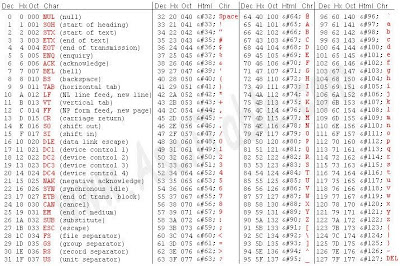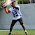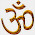### Arduino and RS485 (ENGLISH VERSION)

.

NEWS => Are you interested in CAN Bus with Arduino?  Visit http://secuduino.blogspot.com/

RS485 is a standard serial protocol, with bus topology where you can connect several devices in a very cheap way. Here will be explained how to create a microcontrollers network just with 2 wires.

It is based in a differential couple of wires with which we can get a half-duplex comunication channel. The driver has a configurable input that provides us the possibility to set our transceiver as sender or receiver.

What do we need apart of our Arduino?
• MAX485
• SN75176 (cheaper)

What will be it used for? It is a voltage level converter in order to use our UART as RS485 interface.

Ok, now we are ready to start. RS485 resides in the physical layer based on the OSI Model, so it just tell us about voltages, physic parameters,....

http://www.i-micro.com/pdf/articulos/rs-485.pdfdf
http://www.maxim-ic.com/appnotes.cfm?appnote_number=763&CMP=WP-1
http://www.neoteo.com/rs485-domotica-al-alcance-de-tu-mano-15810.neo-alcance-de-tu-mano-15810.neo
RS485 Oscilloscope Screenshots
http://www.sbc-support.ch/faq/files/files.get.php?ZSESSIONID=n38bphov4ls4cdsvtbd8tl6jb4&fi_index=100179

Then, we need an structural way to send our information. We will use as frames a configuration already created by Fuji in their industrial variators.

Let's take a look at the frame configuration:

• Byte 1: Start Byte ( 0 hexadecimal ).
• Byte 2-3: ASCII Arduino's address.
• Byte 4: Byte ENQ, ACK or NAK (0x05h, 0x06h y 0x15h) .
• Byte 5: ASCII Requested command.
• Byte 6 y 7: ASCII Function number.
• Byte 8: Sign byte (Positive 0x20h y Negative 2D)
• Byte 9-12: ASCII of data bytes (0x00h-0xFFFFh)
• Byte 13: Byte EOT (End of Text) (0x03h)
• Byte 14-15: Checksum (addition from 2nd byte to 13th byte)

With this configuration we have
• 1 Byte indicating the Function Number
• 2 Bytes indicating the sub-function Number

So imagine the possibles combinations!!! For instance:
P01 Request Configuration of PWM 1 with setup data in DATA bytes
And so on!!!! Is not wonderful?

Notice:
- Commands are ASCII Coded
- Control bytes are not ASCII Coded
It is a nice feature due to, in case we receive a 0x00 (thinking it is the start byte), the program will understand it is the starting frame instead of as 0 value, because in this case, it should be sent as ASCII (0x30).

Please take a look at the ASCII Table, even better print it! :P Probably most of us we have a copy pasted in front of us all the time, wrong?
http://www.cs.utk.edu/~pham/ascii_table.jpgLet's see an example:
Imagine we need an arduino(master) which will decide either switch on or switch off a remote lights. Then, it have to send a command on our RS485 network and then other arduino (slave) will interpretate the command and excute it.

Then, the master will follow the switchces state, a and as needed, the master will send the frame requesting switch on/off to the slave called 01. The function will be called D and number 0.(you can set it up as needed). So,
Master sends: Slave(01)-Request execution function(D)-SubFunction(0)
0x00 0x30 0x31 0x05 0x44 0x30 0x30 0x20 0x30 0x30 0x30 0x31 0x03 0x01 0xEE
0x00 0x30 0x31 0x06 0x44 0x30 0x30 0x20 0x30 0x30 0x30 0x31 0x03 0x01 0xEF

The salve's ACK frame has the following configuration(take a look at Byte 4 due to here is specified ACK)

Even better, watch the following video: http://www.youtube.com/watch?v=ABcjU0Ua-d4

Urgh!!!!Sorry but the switcher is just a couple of wires going from VCC to GND! ;)

In order to see the frames flowing on the net, you need just a USB <----> RS232 like FTDI232 or MAX232+MAX485,... If you have an Arduino PCB, notice you can remove the uC from its socket and you'll have an USB <---->Rs232 converter!

If you want to monitorize what is going on , you can use Hyperterminal, or any one that include some useful features as format output, saving options,....for instance we like:
RealTerm
FREE SERIAL TERMINAL MONITOR
Really nice if you want to sniff RS232 frames

In this example we have 3 arduinos connected. The master have 2 switchers used to switch on/off leds connected to each of the slaves.
.

Let's see how it works: http://www.youtube.com/watch?v=S9FSQaToVZ4

Now...THE CODE!

IMPORTANT:
Checksum are done directly in hex instead of convert it to ASCII. Change it could be necessary , to don't have problems. This entry is a howto paper to show you how RS485 works and explain how to implemnt a protocol, but is not 100% debbuged.

MASTER:

```//----------------------------------
//RS 485
//By Igor Real
//24-06-09
//----------------------------------

byte            data;
unsigned long   previous_time;
unsigned long   previous_time2;
byte            times_repeat=5;
byte            times_repeat2=5;

byte            state=0;
byte            state2=0;

#define  pinCONTROL    02

void setup() {

pinMode(13,OUTPUT);
pinMode(pinCONTROL,OUTPUT);
digitalWrite(13,HIGH);
digitalWrite(12,LOW);
pinMode(8,INPUT);
pinMode(9,INPUT);
digitalWrite(pinCONTROL,LOW);
Serial.begin(9600);
Serial.println("Empezamos");
state=0;
state2=0;
}

void loop()
{

state=!state;
times_repeat=0;
}
state2=!state2;
times_repeat2=0;
}

if (times_repeat<4){
Serial.flush();
sendMSG(48,49,68,48,48,32,48,48,48,49);
}else {
sendMSG(48,49,68,48,48,32,48,48,48,48);
}
times_repeat=times_repeat+1;

previous_time=millis();
while (((millis()-previous_time)<500) && (Serial.available()!=15)){
;;
}

if (Serial.available()>=15){
Serial.println("Trama correcta");
if (data==48 && data==49 && data==6){
//ACK
times_repeat=5;
Serial.println("ACK recibido");
}
}
}
}

if (times_repeat2<4){
Serial.flush();
sendMSG(48,50,68,48,48,32,48,48,48,49);
}else {
sendMSG(48,50,68,48,48,32,48,48,48,48);
}
times_repeat2=times_repeat2+1;

previous_time2=millis();
while (((millis()-previous_time2)<500) && (Serial.available()!=15)){
;;
}

if (Serial.available()>=15){
//Serial.println("Trama correcta");
if (data==48 && data==50 && data==6){
//ACK
times_repeat2=5;
//Serial.println("ACK recibido");
}
}
}
}

}

//------------------------
//FUNCIONES
//------------------------

byte  state=0;
byte  cont1=1;
byte  trace_OK=0;

unsigned int checksum;
unsigned int checksum_trace;

while (Serial.available() > 0){

state=1;
checksum_trace=0;
checksum=0;
trace_OK=0;
cont1=1;
}else if (state==1 && cont1<=12){
cont1=cont1+1;
}else if (state==1 && cont1==13){
cont1=cont1+1;
}else if (state==1 && cont1==14){
cont1=cont1+1;
state=0;
if (checksum_trace==checksum){
trace_OK=1;
}else{
trace_OK=0;
}
break;
}
}
return trace_OK;

}

void sendMSG(byte address1,byte address2,byte data_type,byte code1,byte code2,byte Sign,byte data1,byte data2,byte data3,byte data4){

unsigned int checksum_ACK;

UCSR0A=UCSR0A |(1 << TXC0);

digitalWrite(pinCONTROL,HIGH);
delay(1);

Serial.print(0,BYTE);
Serial.print(5,BYTE);
Serial.print(data_type,BYTE);
Serial.print(code1,BYTE);
Serial.print(code2,BYTE);
Serial.print(Sign,BYTE);
Serial.print(data1,BYTE);
Serial.print(data2,BYTE);
Serial.print(data3,BYTE);
Serial.print(data4,BYTE);
Serial.print(3,BYTE);
Serial.print(((checksum_ACK>>8)&255),BYTE);
Serial.print(((checksum_ACK)& 255),BYTE);
while (!(UCSR0A & (1 << TXC0)));
digitalWrite(pinCONTROL,LOW);

}

void sendACK(byte address1,byte address2,byte data_type,byte code1,byte code2,byte Sign,byte data1,byte data2,byte data3,byte data4){

unsigned int checksum_ACK;

UCSR0A=UCSR0A |(1 << TXC0);

digitalWrite(pinCONTROL,HIGH);
delay(1);

Serial.print(0,BYTE);
Serial.print(6,BYTE);
Serial.print(data_type,BYTE);
Serial.print(code1,BYTE);
Serial.print(code2,BYTE);
Serial.print(Sign,BYTE);
Serial.print(data1,BYTE);
Serial.print(data2,BYTE);
Serial.print(data3,BYTE);
Serial.print(data4,BYTE);
Serial.print(3,BYTE);
Serial.print(((checksum_ACK>>8)&255),BYTE);
Serial.print(((checksum_ACK)&255),BYTE);
while (!(UCSR0A & (1 << TXC0)));
digitalWrite(pinCONTROL,LOW);

}

void sendNAK(byte address1,byte address2,byte data_type,byte code1,byte code2,byte Sign,byte data1,byte data2,byte data3,byte data4){

unsigned int checksum_ACK;

UCSR0A=UCSR0A |(1 << TXC0);

digitalWrite(pinCONTROL,HIGH);
delay(1);

Serial.print(0,BYTE);
Serial.print(15,BYTE);
Serial.print(data_type,BYTE);
Serial.print(code1,BYTE);
Serial.print(code2,BYTE);
Serial.print(Sign,BYTE);
Serial.print(data1,BYTE);
Serial.print(data2,BYTE);
Serial.print(data3,BYTE);
Serial.print(data4,BYTE);
Serial.print(3,BYTE);
Serial.print(((checksum_ACK>>8)&255),BYTE);
Serial.print(((checksum_ACK)&255),BYTE);
while (!(UCSR0A & (1 << TXC0)));
digitalWrite(pinCONTROL,LOW);

}

byte hex2num(byte x){

byte result;

if (x>=48 && x<=57){
result=x-48;
}else if (x>=65 && x<=70){
switch(x){
case 65:
result=10;
break;
case 66:
result=11;
break;
case 67:
result=12;
break;
case 68:
result=13;
break;
case 69:
result=14;
break;
case 70:
result=15;
break;
}
}
return result;
}

```

SLAVES:
(you have to change myaddress to 01 or 02)

```//----------------------------------
//RS 485
//By Igor Real
//24-06-09
//----------------------------------

byte  data;
byte  function;
byte  function_code;
byte  state=0;
byte  cont1=1;
byte  trace_OK=0;
unsigned int checksum;
unsigned int checksum_trace;

#define  pinCONTROL    02

void setup() {

pinMode(13,OUTPUT);
pinMode(pinCONTROL,OUTPUT);
digitalWrite(2,LOW);
Serial.begin(9600);
Serial.println("Empezamos");

}

void loop()
{

while (Serial.available() > 0){

//Serial.println("Se ha recibido byte Start");
state=1;
checksum_trace=0;
checksum=0;
trace_OK=0;
cont1=1;
}else if (state==1 && cont1<=12){
cont1=cont1+1;
}else if (state==1 && cont1==13){
cont1=cont1+1;
//Serial.print("Primer Byte Checksum");
//Serial.print(checksum_trace,HEX);
}else if (state==1 && cont1==14){
cont1=cont1+1;
state=0;
//Serial.println("Recibida trama");
//Serial.print("Checksum Trace= ");
//Serial.println(checksum_trace,HEX);
//Serial.print("Checksum= ");
//Serial.println(checksum,HEX);
//Serial.println(checksum,DEC);
//Serial.println("Trama= ");
//Serial.print(data);
//Serial.print(data);
//Serial.print(data);
//Serial.print(data);
//Serial.print(data);
//Serial.print(data);
//Serial.print(data);
//Serial.print(data);
//Serial.print(data);
//Serial.print(data);
//Serial.print(data);
//Serial.println(data);

if (checksum_trace==checksum){
trace_OK=1;

function=data;
function_code=(hex2num(data)<<4)+(hex2num(data));

//Serial.println("TRAZA CORRECTA");
if ((function=='D') && (function_code==0) && data==5){
digitalWrite(13,HIGH);
sendACK(data,data,data,data,data,data,data,data,data,data);
digitalWrite(13,LOW);
sendACK(data,data,data,data,data,data,data,data,data,data);
}
}
}
}else{
//Serial.println("TRAZA INCORRECTA");
sendNAK(data,data,data,data,data,data,data,data,data,data);
}
}

}

}

//------------------------
//FUNCIONES
//------------------------

byte  state=0;
byte  cont1=1;
byte  trace_OK=0;

unsigned int checksum;
unsigned int checksum_trace;

while (Serial.available() > 0){

state=1;
checksum_trace=0;
checksum=0;
trace_OK=0;
cont1=1;
}else if (state==1 && cont1<=12){
cont1=cont1+1;
}else if (state==1 && cont1==13){
cont1=cont1+1;
}else if (state==1 && cont1==14){
cont1=cont1+1;
state=0;
if (checksum_trace==checksum){
trace_OK=1;
}else{
trace_OK=0;
}
break;
}
}
return trace_OK;

}

void sendMSG(byte address1,byte address2,byte data_type,byte code1,byte code2,byte Sign,byte data1,byte data2,byte data3,byte data4){

unsigned int checksum_ACK;

UCSR0A=UCSR0A |(1 << TXC0);

digitalWrite(pinCONTROL,HIGH);
delay(1);

Serial.print(0,BYTE);
Serial.print(5,BYTE);
Serial.print(data_type,BYTE);
Serial.print(code1,BYTE);
Serial.print(code2,BYTE);
Serial.print(Sign,BYTE);
Serial.print(data1,BYTE);
Serial.print(data2,BYTE);
Serial.print(data3,BYTE);
Serial.print(data4,BYTE);
Serial.print(3,BYTE);
Serial.print(((checksum_ACK>>8)&255),BYTE);
Serial.print(((checksum_ACK)& 255),BYTE);
while (!(UCSR0A & (1 << TXC0)));
digitalWrite(pinCONTROL,LOW);

}

void sendACK(byte address1,byte address2,byte data_type,byte code1,byte code2,byte Sign,byte data1,byte data2,byte data3,byte data4){

unsigned int checksum_ACK;

UCSR0A=UCSR0A |(1 << TXC0);

digitalWrite(pinCONTROL,HIGH);
delay(1);

Serial.print(0,BYTE);
Serial.print(6,BYTE);
Serial.print(data_type,BYTE);
Serial.print(code1,BYTE);
Serial.print(code2,BYTE);
Serial.print(Sign,BYTE);
Serial.print(data1,BYTE);
Serial.print(data2,BYTE);
Serial.print(data3,BYTE);
Serial.print(data4,BYTE);
Serial.print(3,BYTE);
Serial.print(((checksum_ACK>>8)&255),BYTE);
Serial.print(((checksum_ACK)&255),BYTE);
while (!(UCSR0A & (1 << TXC0)));
digitalWrite(pinCONTROL,LOW);

}

void sendNAK(byte address1,byte address2,byte data_type,byte code1,byte code2,byte Sign,byte data1,byte data2,byte data3,byte data4){

unsigned int checksum_ACK;

UCSR0A=UCSR0A |(1 << TXC0);

digitalWrite(pinCONTROL,HIGH);
delay(1);

Serial.print(0,BYTE);
Serial.print(15,BYTE);
Serial.print(data_type,BYTE);
Serial.print(code1,BYTE);
Serial.print(code2,BYTE);
Serial.print(Sign,BYTE);
Serial.print(data1,BYTE);
Serial.print(data2,BYTE);
Serial.print(data3,BYTE);
Serial.print(data4,BYTE);
Serial.print(3,BYTE);
Serial.print(((checksum_ACK>>8)&255),BYTE);
Serial.print(((checksum_ACK)&255),BYTE);
while (!(UCSR0A & (1 << TXC0)));
digitalWrite(pinCONTROL,LOW);

}

byte hex2num(byte x){

byte result;

if (x>=48 && x<=57){
result=x-48;
}else if (x>=65 && x<=70){
switch(x){
case 65:
result=10;
break;
case 66:
result=11;
break;
case 67:
result=12;
break;
case 68:
result=13;
break;
case 69:
result=14;
break;
case 70:
result=15;
break;
}
}
return result;
}
```

Now, we will learn how to wire a bus topology:
.
.
Each Arduino or gadget conneted to the net, is wired with an Y welded which comes out 2 connectors from same family different kind(Male/female). The shorter the better. Each connector will have VCC,Sginal A, Singal B and GND. This way, if we want to connect another device onto the net, you need just insert each device or put it at the end. Now is easy understand why we are putting a Y , it's extremely easy connect and disconnect a device from the bus, due to each device has male an female. Is worthy have always a couple of connectors with a end-resistor between Singal A and Signal B RS485 pins in order to "close" the wires' end. If we need add another device is as easy as disconnet the connector with the end-resistor, insert the new device and close again with that connector. Wire VCC and GND is extremely easy, and in future, will make things really easier in possible expansions..... Take care, and twist Signal A and Signal B wires. Please take a loot at next link if needed : http://www.rs-485.com/download/485%20network%20topology.pdf
.
This paper was based on spanish Igor R's one. Thanks Igor R!! Cheers!!!
.
.
Aritz R.
.
.

#### 25 comentarios:

1.This is just as a note for other people:

It took me a while to realize that you need to connect the write enable and read enable lines to the digital pin 2 on the microcontroller.

2.Hi,

Please, find below the pin out of the MAX485 (DIP-8)

PIN-OUT MAX485 --> Arduino
1 -> RO (Receiver Output) to pin 0 (Rx)
2 -> Receive enable to a digital input (ej: pin2)
3 -> Driver enable to a digital (ej:pin2)
4 -> DI (Driver In) to pin 1 (Tx)
5 -> GND to gnd of the arduino
6 -> SIGNAL A (bus RS-485)
7 -> SIGNAL B (bus RS-485)
8 -> Vcc to 5 voltios of the arduino

Regards,

Igor

3.This is great, I've been looking for this for a while! Good detailed explination.

Thanks from NL
Rob

4.Nice tutorial!

question:
is it possible to sent data from the slave to the master in the ACK (in the byte 9 .. 12)?

Floris at deboktor dot nl

5.Hi Floris,
Of course you can. It´s the designer who decides how the protocol works. It´s 100% open.
The RS485 only defines the phisical layer.

Cheers!!

Igor

6.Hi Igor,

I semplified your code, it is easier to mantain it too:

void sendACK_NAK(byte type,byte address1,byte address2,byte data_type,byte code1,byte code2,byte Sign,byte data1,byte data2,byte data3,byte data4){

unsigned int checksum_ACK;

UCSR0A=UCSR0A |(1 << TXC0);

digitalWrite(pinCONTROL,HIGH);
delay(1);

Serial.print(0,BYTE);
Serial.print(type,BYTE);
Serial.print(data_type,BYTE);
Serial.print(code1,BYTE);
Serial.print(code2,BYTE);
Serial.print(Sign,BYTE);
Serial.print(data1,BYTE);
Serial.print(data2,BYTE);
Serial.print(data3,BYTE);
Serial.print(data4,BYTE);
Serial.print(3,BYTE);
Serial.print(((checksum_ACK>>8)&255),BYTE);
Serial.print(((checksum_ACK)&255),BYTE);
while (!(UCSR0A & (1 << TXC0)));
digitalWrite(pinCONTROL,LOW);
}

void sendACK(byte address1,byte address2,byte data_type,byte code1,byte code2,byte Sign,byte data1,byte data2,byte data3,byte data4){
}

void sendNAK(byte address1,byte address2,byte data_type,byte code1,byte code2,byte Sign,byte data1,byte data2,byte data3,byte data4){
}

1.Giangi,
Corrected sendNAK should have been as below (because NAK = 0x15 = 21:

void sendNAK(byte address1,byte address2,byte data_type,byte code1,byte code2,byte Sign,byte data1,byte data2,byte data3,byte data4){
}

7.And this one too:

byte hex2num(byte x){
byte result;

if (x>=48 && x<=57){ //numbers 0-9
result=x-48;
}else if (x>=65 && x<=70){ //A-F
result=x-55; //A=10, B=11, …,F=15
}
return result;
}

8.Igor I think there is a bug in the original sendNAK function.
Should the row
be corrected in
?
Notice the 21 value (0x15h) instead of 6 because you wrote ENQ, ACK or NAK (0x05h, 0x06h y 0x15h).

In this case the code is much simplier because you can merge the sendMSG code too:

void sendMSG(byte type, byte address1,byte address2,byte data_type,byte code1,byte code2,byte Sign,byte data1,byte data2,byte data3,byte data4){

unsigned int checksum_ACK;

UCSR0A=UCSR0A |(1 << TXC0);

digitalWrite(pinCONTROL,HIGH);
delay(1);

Serial.print(0,BYTE);
Serial.print(type,BYTE);
Serial.print(data_type,BYTE);
Serial.print(code1,BYTE);
Serial.print(code2,BYTE);
Serial.print(Sign,BYTE);
Serial.print(data1,BYTE);
Serial.print(data2,BYTE);
Serial.print(data3,BYTE);
Serial.print(data4,BYTE);
Serial.print(3,BYTE);
Serial.print(((checksum_ACK>>8)&255),BYTE);
Serial.print(((checksum_ACK)&255),BYTE);
while (!(UCSR0A & (1 << TXC0)));
digitalWrite(pinCONTROL,LOW);
}

void sendACK(byte address1,byte address2,byte data_type,byte code1,byte code2,byte Sign,byte data1,byte data2,byte data3,byte data4){
}

void sendNAK(byte address1,byte address2,byte data_type,byte code1,byte code2,byte Sign,byte data1,byte data2,byte data3,byte data4){
}

9.Good points Giangi!! Please feel free to send me your improvements and I can publish them here.

Thanks!

;)

10.Hi,

I'm wondering if there won't be any problems with addresses if I set master to 01 and slaves as u wrote 01 and 02. Shouldn't be for ex. master 01, slave_1 02, and slave_2 03 ??

I hope it isn't a stupid question - I'm just starting with it

cheers.

11.Hi,
can send me a schematic to build this? Where to put the resistor and where the jumper?

Thank You

Andreas

12.Hi,
Could you please post a schematic of how you wired the led and the pushbutton?

Thank's,
Cristian

13.I've tried to implement the same circuit, but I'm facing a problem to use the Arduino as USB->Serial converter. I just receive datas in the PC, but I'm not able to send datas from it.
Could you help me?
Thanks

14.Hello, I'd like to know, which are the parts of the whole message that I can use to communicate the commands?

15.Hello Well done for this nice job ! ! !

I would like to ask if it is possibly to make a library so less code need to use in the IDE.

Thank you.

16.Noob question:

I want to connect several sensors to an Arduino, but each sensor will be far from it, like 20 to 30 meters. Is it possible to send the sensor data (ultrasound/temp) via RS485?

Thanks,

Alvaro

1.Yes it´s possible.

Regards,

Igor

17.Is it possible to have the master turn on a light at the slave. The slave to turn on a light at the master. And a button at the master to turn off both lights?
Thanks.

18.Hi, I'm having some trouble understanding the two following lines of code.

"UCSR0A=UCSR0A |(1 << TXC0);" as well as "while (!(UCSR0A & (1 << TXC0)));"

I have recently finished a project using the MAX485 chip using Arduinos as 1 master and 3 slaves transmitting about 20 metres. I ran into an issue where I was required to add a 50ms delay between pulling the transmit pin high and sending the serial data (in my case chars). If the delay was any shorter then the slaves would only read gibberish.
There was also the issue that when two of the slaves were transmitting at the same time, the master would also just see gibberish. Although I'm not sure if that was a timing issue or something else.
Any insight you could give me would be much appreciated.
Thanks :)

19.Hola Igor he estado viendo tus tutoriales el de ingles y español, quiero hacer un sistemas de bus de datos a dos hilos pero mi esquema va Arduino-Rs485-sensor como hago que desde el arduino pida el dato del sensor. 2. corri tu codigo y me marca este error.

byte, byte, byte, byte, byte, byte, byte)':
sketch_sep11a:185: error: 'BYTE' was not declared in this scope

Desde Arduino 1.0, la palabra clave 'BYTE' ya no está soportada en
Serial.print(var, BYTE). Por favor utiliza Serial.write() en su lugar.

me podrias ayudar¿ Gracias

1.Just delete the "BYTE" thing. Arduino nowadays sends the serial data byte like so if you say BYTE is like a double declaration.

20.Hello sir,
I'm new in arduino and rs485. I have a Siedle Automation System in my home and I want to make an upgrade to it. Siedle System has a card reader and a central processing unit that uses RS485 protocol at 9600 baud rate to comunicate with the multiple card readers. I used your slave code to get in the bus and read data. I've seen different reads at different cards, wich is what I want to do, making an if function to determine wich card is wich and to actuate rellays in case of some of the cards.

This is the log i recive from the serial reading:

Am primit codul:
A8 3 6 2 40 2D 11 82 3 6 checksum:
11522
01683152644517130363831229Am primit byte-ul de start: 0
Am primit bit:?3

That long number is the number i need to pull out for the if thing, but I can't undestand where it comes from.
Here is the code: (what I've changed from )
while (Serial.available() > 0){

Serial.print("Am primit byte-ul de start:");
Serial.print("\t");
state=1;
checksum_trace=0;
checksum=0;
trace_OK=0;
cont1=1;
}else if (state==1 && cont1<=12){
cont1=cont1+1;
}else if (state==1 && cont1==13){
cont1=cont1+1;
//Serial.print("Primer Byte Checksum");
//Serial.print(checksum_trace,HEX);
}else if (state==1 && cont1==14){
cont1=cont1+1;
state=0;
Serial.print("Am primit bit:?");
Serial.println("Am primit codul:");
//Serial.print("Checksum Trace= ");
//Serial.println(checksum_trace,HEX);
//Serial.print("Checksum= ");
//Serial.println(checksum,HEX);
//Serial.println(checksum,DEC);
Serial.println("Datele= ");
Serial.print(data, HEX);
Serial.print("\t");
Serial.print(data, HEX);
Serial.print("\t");
Serial.print(data, HEX);
Serial.print("\t");
Serial.print(data, HEX);
Serial.print("\t");
Serial.print(data, HEX);
Serial.print("\t");
Serial.print(data, HEX);
Serial.print("\t");
Serial.print(data, HEX);
Serial.print("\t");
Serial.print(data, HEX);
Serial.print("\t");
Serial.print(data, HEX);
Serial.print("\t");
Serial.print(data, HEX);
Serial.print("\t");

Thank you very much.

21.Hi, I’m Mehul, the author of this blog. For me, learning is a life long adventure. Moreover, learning anything in this era is not impossible: if you will it, you can learn and do anything.

This is how my interest in programming and electronics materialized…

22.Hi, great tutaorial, thanks.
Do you know what is the latency between the button press and the led on ?